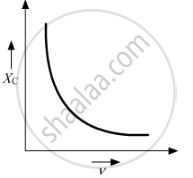# Draw a Graph to Show Variation of Capacitive-reactance with Frequency in an A.C. Circuit. - Physics

Draw a graph to show variation of capacitive-reactance with frequency in an a.c. circuit.

#### Solutionwhere XC is the capacitive-reactance and is the frequency in an a.c circuit.

Concept: Reactance and Impedance
Is there an error in this question or solution?